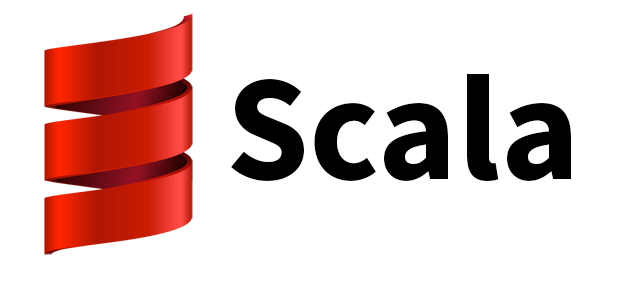In Scala function parameters are passed by value, that means that the parameters are evaluated before are passed to the function.

Sometimes this is not the best behaviour. Fortunately, Scala lets you pass parameters by name if you need it

Let’s see an example

We’re going to use the Scala REPL to see the example.

First of all, we define a function

```scala> def optional(x: Int): Int = {
|   println("Optional")
|   x
| }
optional: (x: Int)Int
```

An we call it to see what this function does

```scala> optional(3)
Optional
res0: Int = 3
```

The function prints Optional in the console and returns the parameter we pass to it

Let’s define another function

```scala> def myFunction(y: Int, f: Int => Int) = {
|   if (y > 0)
|     println(f(y))
|   println("myFunction")
| }
myFunction: (y: Int, f: Int => Int)Unit
```

Let’s see it in action

```scala> myFunction(3, optional)
Optional
3
myFunction

scala> myFunction(-3, optional)
myFunction
```

The function prints the returned value from our `optional` function if the `y` parameter is greater than 0 and the prints myFunction

Let’s do a little change to our function

```scala> def myFunction(y: Int, x: Int) = {
|   if (y > 0)
|     println(x)
|   println("myFunction")
| }
myFunction: (y: Int, x: Int)Unit

scala> myFunction(3, 5)
5
myFunction

scala> myFunction(-3, 5)
myFunction
```

Now the function takes two parameters and if the parameter `y` is greater than 0 it prints the value of the parameter `x` and the prints myFunction

But what happens is we want to use the value of our `optional` function to print it. Let’s see what happens

```scala> myFunction(3, optional(5))
Optional
5
myFunction

scala> myFunction(-3, optional(5))
Optional
myFunction
```

As we can see the `optional` function is called whether the value is printed or not. That’s because Scala is evaluating the `optional` function to pass the value as a parameter

We can modify this changing the function definition like this

```scala> def myFunction(y: Int, x: => Int) = {
|   if (y > 0)
|     println(x)
|   println("myFunction")
| }
myFunction: (y: Int, x: => Int)Unit
```

Note that we are adding a `=>` before the type definition of the `x` parameter. This is telling Scala to evaluate the parameter only when we need it. Let’s see how our output changes

```scala> myFunction(3, optional(5))
Optional
5
myFunction

scala> myFunction(-3, optional(5))
myFunction
```

Let’s do another change to see what happens if we need our value more than once

```scala> def myFunction(y: Int, x: => Int) = {
|   if (y > 0) {
|     println(x)
|     println(x)
|   }
|   println("myFunction")
| }
myFunction: (y: Int, x: => Int)Unit

scala> myFunction(3, optional(5))
Optional
5
Optional
5
myFunction
```

As we see the parameter is evaluated both times so this code will be optimal if we pass our parameter by value. You must decide when you want to use parameter by value and when parameter by name in your code

comentarios